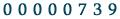# Around the orbit equivalence theory of the free group, cost and ℓ2-Betti numbers

## D. Gaboriau

Initially: preparatory and personal notes for various Masterclasses on Ergodic Theory, Measure Equivalence, Cost and L^2 Betti numbers at various places.
Regular additions (2016, 2018, 2019, 2020).
Trying to keep track of new developments in cost theory.
Document in rough draft form.

## Abstract

The goal of this series of lectures is to present an overview of the theory of orbit equivalence, with a particular focus on the probability measure preserving actions of the free groups. I will start by giving the basis of the theory of orbit equivalence and explain the theory of cost. In particular, prove such statements as the induction formula and the computation of the cost of free actions of some countable groups, including free groups. This will be related to the fundamental group of equivalence relations. I intend to present Abert-Nikolov theorem relating the cost of profinite actions to the rank gradient of the associated chain of subgroups. I will consider a recent result of F. Le Maître establishing a perfect connection between the cost of a probability measure preserving action with the number of topological generators of the associated full group. I shall also discuss the number of non orbit equivalent actions of countable groups.

* Earlier versions Copenhagen Lectures